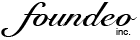# CFSCRIPT ColdFusion Cheat Sheet

#### FOR Loop

```for (i=1;i LTE ArrayLen(array);i=i+1) {
WriteOutput(array[i]);
}
```

#### User Defined Function (UDF)

```function funky(mojo) {
var dojo = 0;
if (arguments.mojo EQ dojo) {
return "mojo";
}
else { return "no-mojo"; }
}
```

#### Switch Case

```switch(fruit) {
case "apple":
WriteOutput("I like Apples");
break;
case "orange":
WriteOutput("I like Oranges");
break;
default:
WriteOutput("I like fruit");
}
```

#### If / Else If / Else

```if (fruit IS "apple") {
WriteOutput("I like apples");
}
else if (fruit IS "orange") {
WriteOutput("I like oranges");
}
else {
WriteOutput("I like fruit");
}
```

#### While Loop

```x = 0;
while (x LT 5) {
x = x + 1;
WriteOutput(x);
}
//OUTPUTS 12345
```

#### Do / While Loop

```x = 0;
do {
x = x+1;
WriteOutput(x);
} while (x LTE 0);
// OUTPUTS 1
```

#### Assign a variable

`foo = "bar";`

#### Single Line Comment

`mojo = 1; //THIS IS A COMMENT`

#### CFScript Component CF9+

```component extends="Fruit" output="false" {

property name="variety" type="string";

public boolean function isGood() {
return true;
}

private void eat(required numeric bites) {
//do stuff
}
}
```

#### Try / Catch / Throw / Finally / Rethrow

```try {
throw(message="Oops", detail="xyz"); //CF9+
} catch (any e) {
WriteOutput("Error: " & e.message);
rethrow; //CF9+
} finally { //CF9+
WriteOutput("I run even if no error");
}
```

#### FOR IN Loop (Structure)

```struct = StructNew();
struct.one = "1";
struct.two = "2";
for (key in struct) {
WriteOutput(key);
}
//OUTPUTS onetwo
```

#### Multiline Comment

```/* This is a comment
that can span
multiple lines
*/
```

#### FOR IN Loop (Array) CF9.0.1+

```cars = ["Ford","Dodge"];
for (car in cars) {
WriteOutput(car);
}
//OUTPUTS FordDodge
```

#### FOR IN Loop (Query) CF10+

```cars = QueryNew("make,model",
"cf_sql_varchar,cf_sql_varchar",
[["Ford", "T"],["Dodge","30"]]);
for (car in cars) {
WriteOutput("Model " & car.model);
}
//OUTPUTS Model TModel 30
```

#### CFInclude CF9+

`include "template.cfm";`

#### Transactions CF9+

```transaction {
//do stuff
if (good) {
transaction action="commit";
else {
transaction action="rollback";
}
}
```

#### Struct Literal CF8+

`product = {id=1, name="Widget"};`

#### Array Literal CF8+

`fruit = ["apples", "oranges"];`
Tip: Don't forget to include a semicolon `;` after each statement!

Questions, comments, criticism, or requests can be directed Here